# 基于 DolphinDB 机器学习的出租车行程时间预测

04/20 14:29

DolphinDB 集高性能时序数据库与全面的分析功能为一体，可用于海量结构化数据的存储、查询、分析、实时计算等，在工业物联网场景中应用广泛。本文以纽约出租车行程时间预测为例，介绍如何使用 DolphinDB 训练机器学习模型，并进行实时数据的预测为基于智能网联汽车的车联网企业提供基于机器学习方法的即时预测方案

## 1. 概要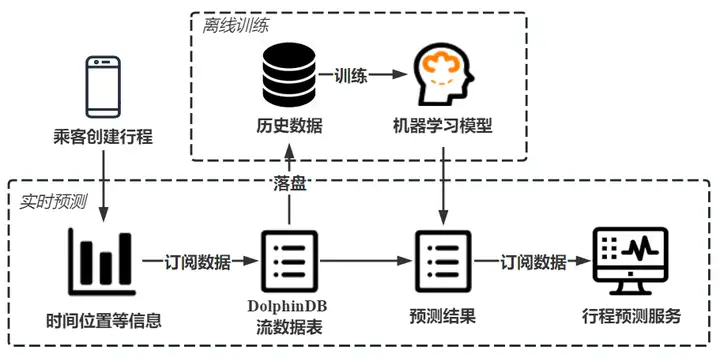## 2. 数据介绍

### 2.2 数据特征

id SYMBOL 行程的唯一标识 id2875421
vendor_id INT 行程记录提供商代码 2
pickup_datetime DATETIME 出租车计价器开启时间 2016/3/14 17:24:55
dropoff_datetime DATETIME 出租车计价器关闭时间 2016/3/14 17:32:30
passenger_count INT 乘客数量 1
pickup_longitude DOUBLE 出租车计价器开启位置经度 -73.98215484619139
pickup_latitude DOUBLE 出租车计价器开启位置纬度 40.76793670654297
dropoff_longitude DOUBLE 出租车计价器关闭位置经度 -73.96463012695312
dropoff_latitude DOUBLE 出租车计价器关闭位置纬度 40.765602111816406
store_and_fwd_flag CHAR 标识来源是否为存储的历史数据 N
trip_duration INT 行程时间（按秒计） 455

DolphinDB 支持 loadText 方法读取 csv 等数据存储文件到内存表，用户可以 schema 函数获取表的特征信息。DolphinDB 也支持使用 SQL 语句完成数据的查询。

train = loadText("./taxidata/train.csv")
train.schema().colDefs
select count(*) from train
select top 5 * from train

## 3. 模型构建

### 3.1 数据预处理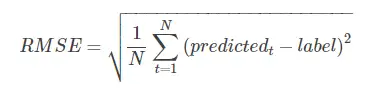RMSE

DolphinDB 提供多种计算函数，可以帮助用户快速实现数据处理。DolphinDB 提供 isNull() 方法用于判断空值，配合 sum() 等聚合函数使用可以快速完成整表数据的查询；提供类似于条件运算符的 iif() 方法简化 if-else 语句；date()weekday()hour() 等方法可以提取时间、日期数据的不同特征，简洁高效；类似于 Python 等编程语言，DolphinDB 支持方括号([])索引，简化了表的查找、更新和插入。

sum(isNull(train))  // 0，不含空值
trainData[store_and_fwd_flag_int] = iif(trainData[store_and_fwd_flag] == 'N', int(0), int(1)) // 将字符N/Y转化为0/1值
trainData[pickup_date] = date(trainData[pickup_datetime]) // 日期
trainData[pickup_weekday] = weekday(trainData[pickup_datetime]) // 星期*
trainData[pickup_hour] = hour(trainData[pickup_datetime]) // 小时
trainData[log_trip_duration] = log(double(trainData[trip_duration]) + 1)// 对行程时间取对数，log(trip_duration+1)
select max(trip_duration / 3600) from trainData // 训练集上最大行程时间为979h

### 3.2 位置信息主成分分析（PCA）

DolphinDB PCA 返回的结果是一个字典，包含 components、explainedVarianceRatio、singularValues 三个键，分别代表对应大小为 size(colNames)*k 的主成分分析矩阵、前k个主成分每个特征的方差贡献率、主成分方差（协方差矩阵特征值）。可通过主成分分析矩阵转换待处理数据，详见 Scikit-Learn PCA.transform()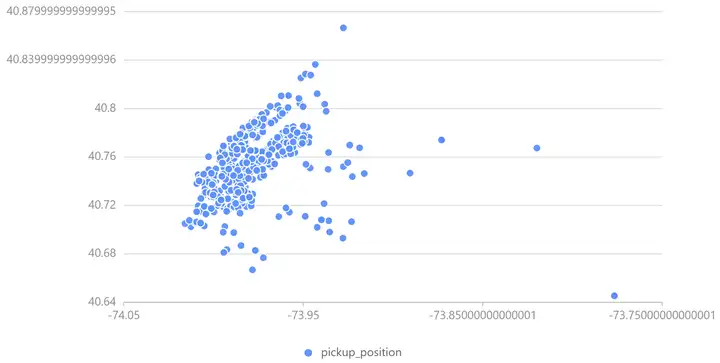PCA前上客位置信息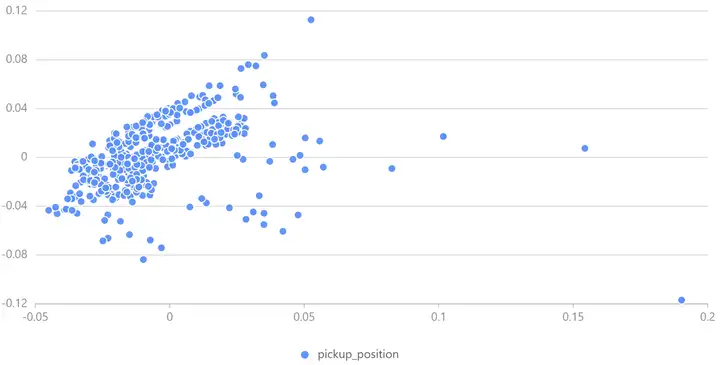PCA 后上客位置信息

pca() 接收一个或多个数据源为参数，对指定列中的数据进行主成分分析，用户可通过 table() 方法创建内存表，用于 PCA；DolphinDB 也提供了 dot()repmat() 等矩阵乘法、矩阵堆叠方法，用户可使用内置函数快速完成矩阵运算，处理位置信息。

PCApara = table(trainData[pickup_latitude] as latitude, trainData[pickup_longitude] as longitude)
pca_model = pca(sqlDS(<select * from PCApara>)) // 使用PCA计算数据集属性
pca_trainpick = dot((matrix(trainPickPara) - repmat(matrix(avg(trainPickPara)), train_n, 1)), pca_model.components) // transform
trainData[pca_trainpick_0] = flatten(pca_trainpick[:, 0])

DolphinDB 提供了plot函数供数据可视化。用户可通过chartType指定图表类型，详见plot — DolphinDB 2.0 documentation

x = select top 1000 pca_trainpick_1 from trainData
y = flatten(matrix(select top 1000 pca_trainpick_0 from trainData))
plot(x, y, chartType=SCATTER)

### 3.3 位置信息聚类（KMeans）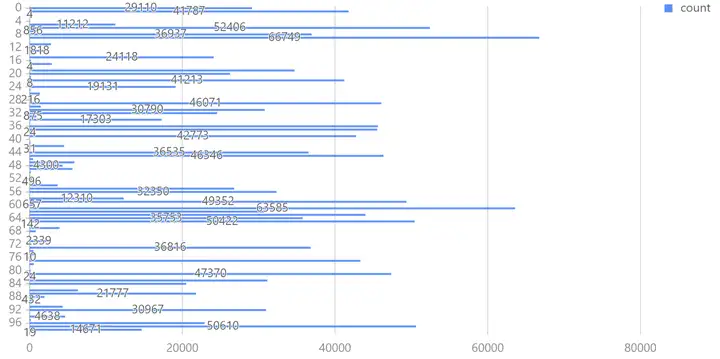KMeans 聚类结果

kmeans() 接收一个表作为训练集。对于机器学习函数生成的模型，DolphinDB 提供 saveModel 方法将模型保存到本地文件中用于后续预测，用户可指定服务器端输出文件的绝对路径或相对路径；DolphinDB 也提供了 predict 方法，调用已训练的特定模型对相同表结构的测试集数据进行预测。

kmeans_set = PCApara[rand(size(PCApara)-1, 500000)] // 随机选取500000数据用于聚类
kmeans_model = kmeans(kmeans_set, 100, maxIter=100, init='k-means++') // KMeans++
saveModel(kmeans_model, "./taxidata/KMeans.model") // 保存模型训练结果
trainData['pickup_cluster'] = kmeans_model.predict(select pickup_latitude, pickup_longitude from trainData)

saveModel 和 predict 函数的使用方法可以参考：

### 3.4 新特征构建

// 两经纬度点距离、两个经纬度之间的 Manhattan 距离、两个经纬度之间的方位信息
trainData['distance_haversine'] = haversine_array(trainData['pickup_latitude'], trainData['pickup_longitude'], trainData['dropoff_latitude'], trainData['dropoff_longitude'])
trainData['distance_dummy_manhattan'] = dummy_manhattan_distance(trainData['pickup_latitude'], trainData['pickup_longitude'], trainData['dropoff_latitude'], trainData['dropoff_longitude'])
trainData['direction'] = bearing_array(trainData['pickup_latitude'], trainData['pickup_longitude'], trainData['dropoff_latitude'], trainData['dropoff_longitude'])
// 按时间、聚类等信息处理速度、行程时间，产生新特征
for(gby_col in ['pickup_hour', 'pickup_date', 'pickup_week_hour', 'pickup_cluster', 'dropoff_cluster']) {
for(gby_para in ['avg_speed_h', 'avg_speed_m', 'log_trip_duration']) {
gby = groupby(avg, trainData[gby_para], trainData[gby_col])
gby.rename!(avg_ + gby_para, gby_para + '_gby_' + gby_col)
trainData = fj(trainData, gby, gby_col)
testData = fj(testData, gby, gby_col)
}
trainData.dropColumns!(gby + gby_col)
}

### 3.5 模型训练（XGBoost）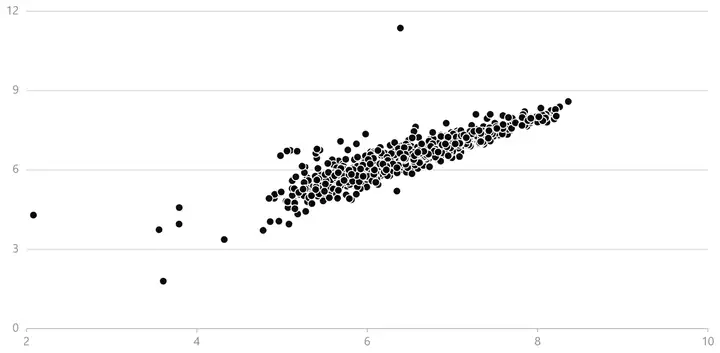DolphinDB 提供了 XGBoost 插件实现模型训练及预测，使用前需要下载插件到指定路径并加载 XGBoost 插件。DolphinDB XGBoost 插件使用详见 xgboost/README_CN.md · dolphindb/DolphinDBPlugin - Gitee

xgb_pars = {'min_child_weight': 50, 'eta': 0.3, 'colsample_bytree': 0.3, 'max_depth': 10,
'subsample': 0.8, 'lambda': 1., 'nthread': 4, 'booster' : 'gbtree', 'silent': 1,
'eval_metric': 'rmse', 'objective': 'reg:linear', 'nthread': 48} // xgb 参数设置
xgbModel = xgboost::train(ytrain, train, xgb_pars, 60) // 训练模型
yvalid_ = xgboost::predict(xgbModel, valid) // 使用模型进行预测

### 3.6 模型评价

Python Scikit-Learn 也是主流的机器学习库之一，本文在相同环境下使用 Python 对同一数据集进行训练，PCA、KMeans++、XGBoost 训练耗时如下表所示：

PCA 0.325s 0.396s
KMeans++ 45.711s 104.568s
XGBoost 57.269s 74.289s

DolphinDB 与 Python 训练模型在验证集上误差如下表所示：

DolphinDB Python
RMSE 0.390 0.394

## 4. 行程时间实时预测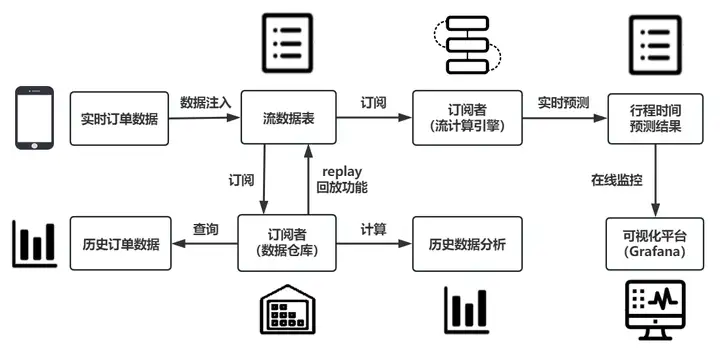DolphinDB 流数据处理框架

### 4.1 场景描述

DolphinDB 流数据模块采用发布-订阅-消费的模式，流数据首先注入流数据表中，通过流表来发布数据，第三方应用可以通过 DolphinDB 脚本或 API 订阅及消费流数据。

### 4.2 实时数据模拟及预测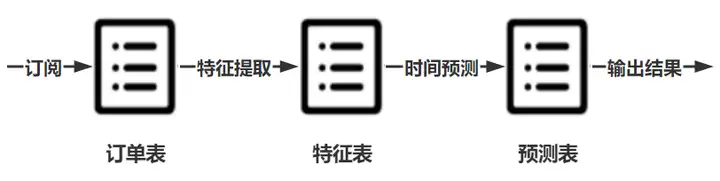// 订阅订单信息表，数据从订单表流向特征表
subscribeTable(tableName="orderTable", actionName="orderProcess", offset=0, handler=process{traitTable, hisData}, msgAsTable=true, batchSize=1, throttle=1, hash=0, reconnect=true)
// 订阅特征表，数据从特征表流向预测表
subscribeTable(tableName="traitTable", actionName="predict", offset=0, handler=predictDuration{predictTable}, msgAsTable=true, hash=1, reconnect=true)
// 回放历史数据，模拟实时产生的生产数据
submitJob("replay", "trade",  replay{inputTables=data, outputTables=orderTable, dateColumn=pickup_datetime, timeColumn=pickup_datetime, replayRate=25, absoluteRate=true, parallelLevel=1})

### 4.3 Grafana 实时监控

Grafana 是一个用于时序数据动态可视化的数据展示工具，DolphinDB 提供了 Grafana 的数据接口，用户可在 Grafana 面板上编写查询脚本与 DolphinDB 进行交互，实现 DolphinDB 时序数据的可视化，并 Web 端进行实时数据分析，详见 README.zh.md · dolphindb/grafana-datasource - Gitee

• Query 1：展示当日出行订单预估到达时间及预估行程时间
select id as ID, pickup_datetime as pickup_time, (pickup_datetime+int((exp(duration)-1))) as arrival_time,  (exp(duration)-1)/60 as duration from predictTable
where date(predictTable.pickup_datetime) == date(select max(pickup_datetime) from predictTable) 
• Query 2：统计当日累计订单数及累计乘客数
select count(*) from predictTable
where date(predictTable.pickup_datetime) == date(select max(pickup_datetime) from predictTable)
select sum(passenger_count) from predictTable
where date(predictTable.pickup_datetime) == date(select max(pickup_datetime) from predictTable)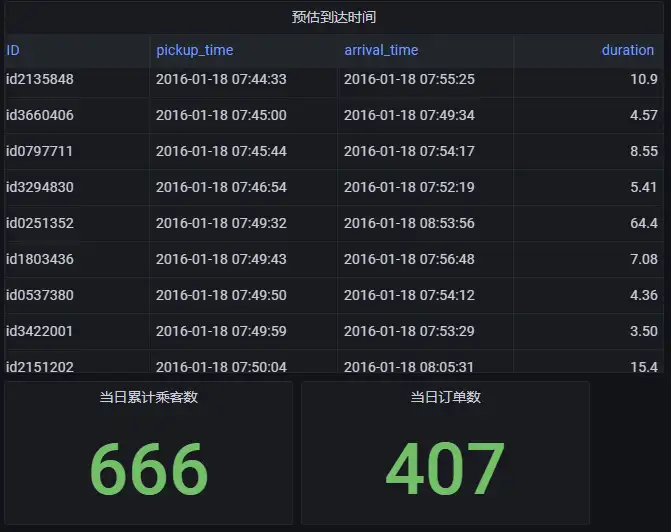• Query 3：统计当日乘客上车位置
select pickup_latitude as latitude, pickup_longitude as longitude from predictTable
where date(predictTable.pickup_datetime) == date(select max(pickup_datetime) from predictTable)• Query 4：统计当日不同时刻订单出行耗时
select pickup_datetime, (exp(duration)-1)/60 as duration from predictTable
where date(predictTable.pickup_datetime) == date(select max(pickup_datetime) from predictTable)### 4.4 数据持久化

db = database("dfs://taxi")
if(existsTable("dfs://taxi", "newData")) { dropTable(db, "newData") }
db.createPartitionedTable(table=table(1:0, orderTable.schema().colDefs.name, orderTable.schema().colDefs.typeString), tableName=newData, partitionColumns=pickup_datetime, sortColumns=pickup_datetime, compressMethods={datetime:"delta"})
subscribeTable(tableName="orderTable", actionName="saveToDisk", offset=0, handler=loadTable("dfs://taxi", "newData"), msgAsTable=true, batchSize=100000, throttle=1, reconnect=true)

## 6. 附录

### 6.1 测试环境

• 操作系统：Linux version 3.10.0-1160.el7.x86_64
• CPU：Intel(R) Xeon(R) Silver 4214 CPU @2.20GHz 48核
• 内存：188G
• 软件版本：
• DolphinDB：2.00.9
• Python3：3.7.12
• Scikit-Learn：1.0.2
• XGBoost：1.6.2

### 6.2 模型代码

DolphinDB 模型训练代码：taxiTrain.dos

DolphinDB 流数据预测代码： taxiStream.dos

Python 模型训练代码： taxiTrain.py0 评论
0 收藏
0